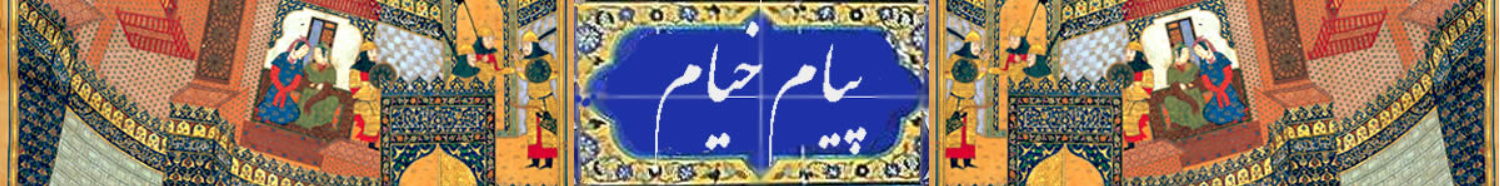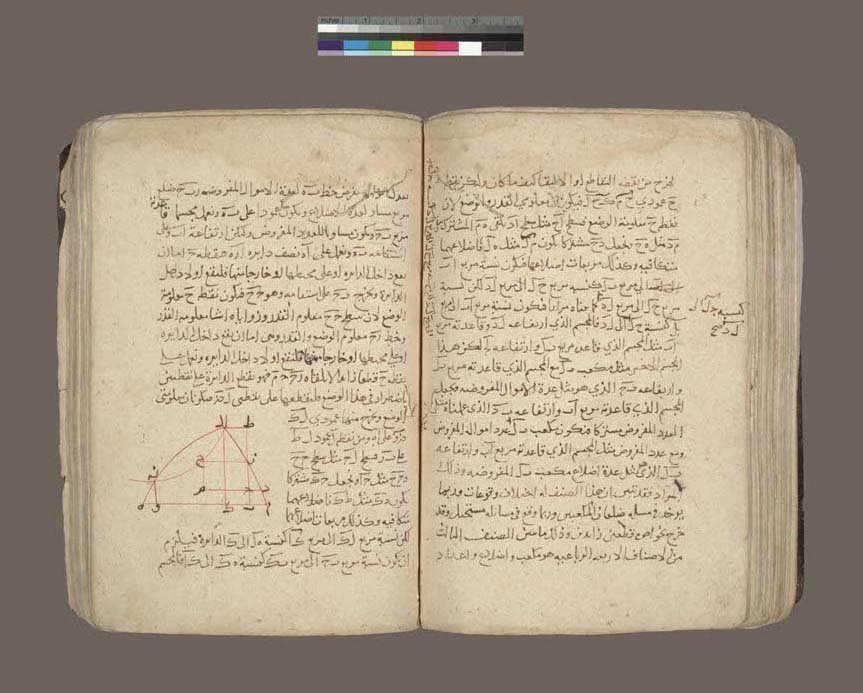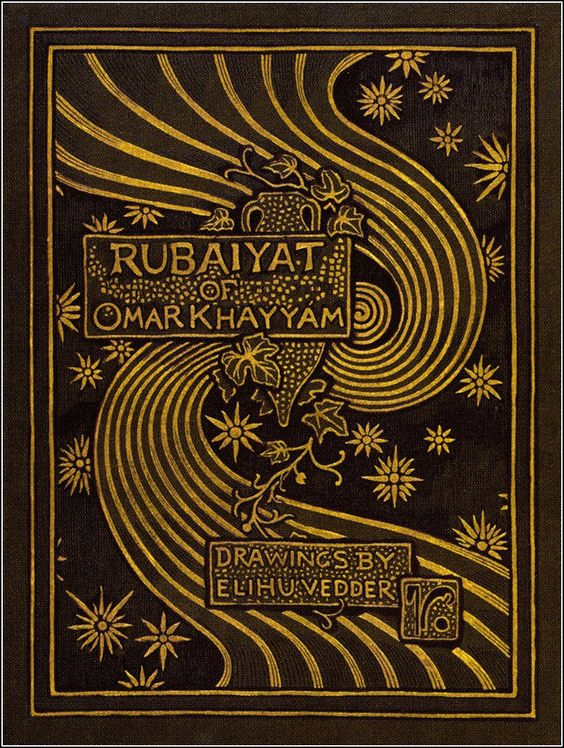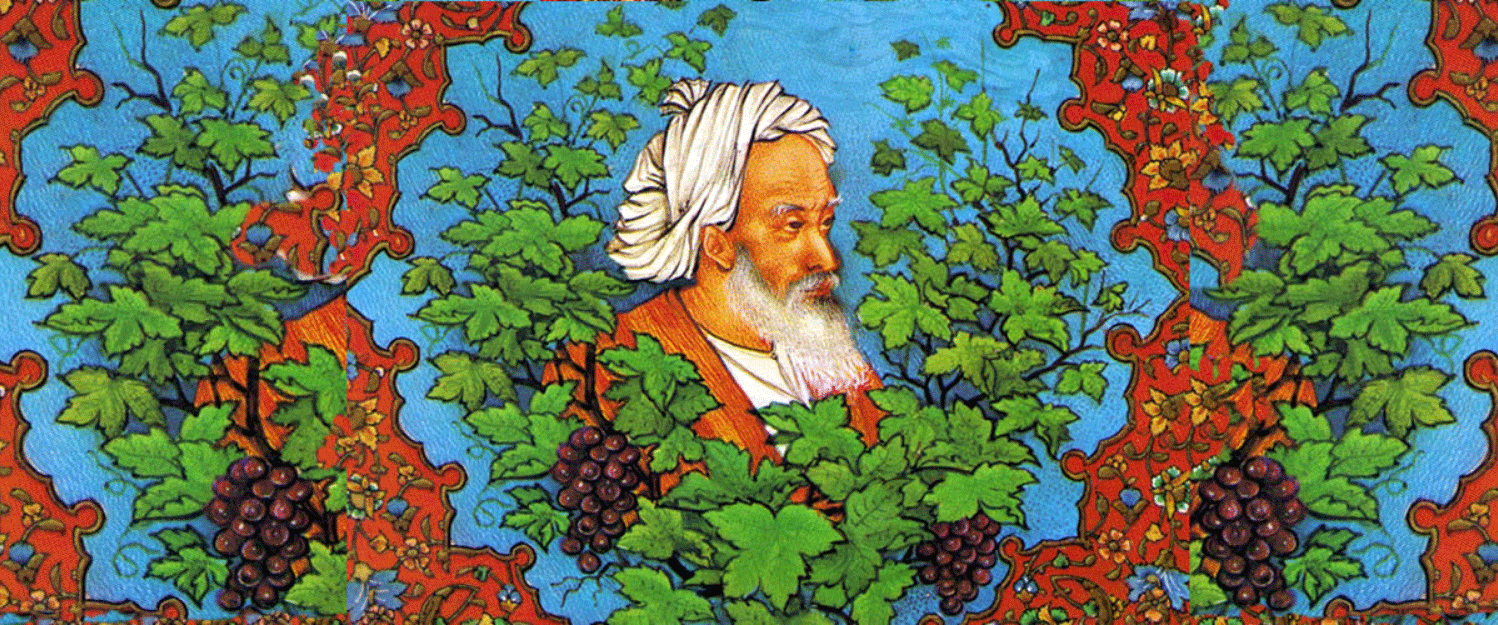# The Rubáiyát – Omar KhayyámThis is a page from a manuscript of the Algebra (Maqalah fi al-jabra wa-al muqabalah) of Omar Khayyam (1048-1131). This work is known for its solution of the various cases of the cubic equation by finding the intersections of appropriately chosen conic sections. On this page, Omar is discussing the case “a cube, sides and numbers are equal to squares”, or, in modern notation, x3 + cx + d = bx2. The two conics whose intersection provides the solution are a circle and a hyperbola. In the case illustrated, these curves intersect twice, thus providing two (positive) solutions of the given cubic equation. Khayyam even provides a problem which leads to this case: Divide ten into two parts so that the sum of the squares of the parts together with the quotient of the division of the greater by the smaller be seventy-two. For more details, see pp. 90ff of Daoud Kasir, The Algebra of Omar Khayyam (New York: Teachers College Press, 1931) or pp. 144ff of R. Rashed and B. Vahabzadeh, Omar Khayyam, the Mathematician (New York: Bibliotheca Persica Press, 2000).

This particular manuscript was copied in the thirteenth century in Lahore, India. Among the other fourteen works contained in the volume are two by Sharaf al-Din al-Tusi (1135-1213) on determining vertical heights of objects and a treatise by ibn al-Haytham (965-1039) on the astrolabe.